# 神经网络变得轻松（第四部分）：循环网络

### 内容

• 概述
• 1. 循环神经网络的显著特征
• 2. 循环网络训练原则
• 3. 构建一个循环神经网络
• 3.1. 类的初始化方法
• 3.2. 前馈
• 3.3. 误差梯度计算
• 3.4. 更新权重
• 4. 测试
• 结束语
• 链接
• 本文中用到的程序

### 1. 循环神经网络的显著特征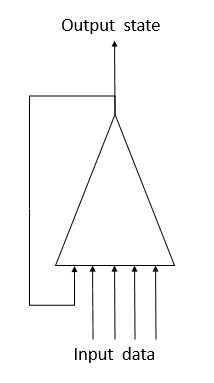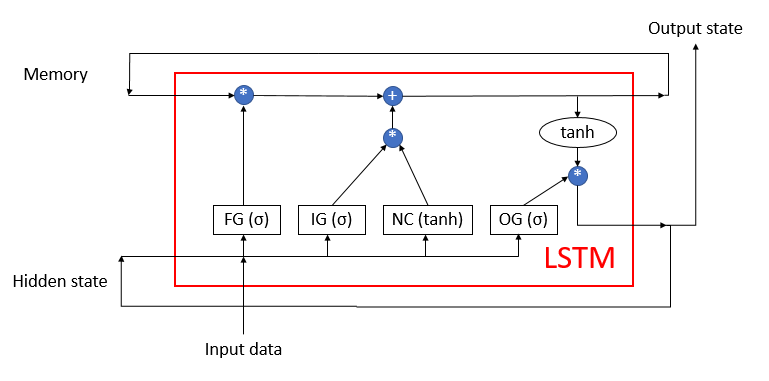LSTM 很难被称为神经元。 它已经是含有3 个输入通道和 3 个输出通道的神经网络。 仅使用两个通道（一个用于输入，另一个用于输出）与外界交换数据。 其余四个通道成对封闭，以便循环进行信息交换（记忆隐藏状态）。

LSTM 模块包含两个主要数据流，这些数据流由 4 个完全连接神经层互连。 所有神经层都包含相同数量的神经元，等于输出流和记忆流的大小。我们来更详尽地研究该算法。

• 输入数据描述系统的当前状态。
• 记忆隐藏状态接收上一个状态。

• 新内容 — 含有双曲正切作为激活函数的完全连接神经层。 它将接收到的信息规范化为 -1 到 1。
• 输入门户 — 含有希格玛形作为激活函数的完全连接神经层。 它类似于“遗忘门户”，并判定要记忆的新数据。

### 2. 循环网络训练原则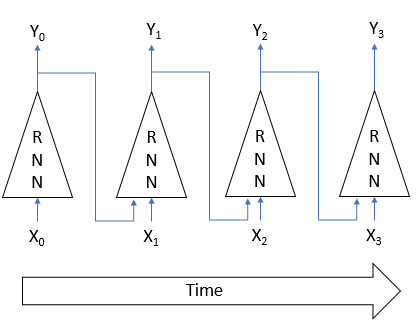### 3. 构建一个循环神经网络

```class CNeuronLSTM    :  public CNeuronProof
{
protected:
CLayer            *ForgetGate;
CLayer            *InputGate;
CLayer            *OutputGate;
CLayer            *NewContent;
CArrayDouble      *Memory;
CArrayDouble      *Input;
//---
virtual bool      feedForward(CLayer *prevLayer);
virtual bool      updateInputWeights(CLayer *&prevLayer);
virtual bool      updateInputWeights(CLayer *gate, CArrayDouble *input_data);
virtual bool      InitLayer(CLayer *layer, int numOutputs, int numOutputs);
virtual CArrayDouble *CalculateGate(CLayer *gate, CArrayDouble *sequence);

public:
CNeuronLSTM(void);
~CNeuronLSTM(void);
virtual bool      Init(uint numOutputs,uint myIndex,int window, int step, int units_count);
//---
virtual CLayer    *getOutputLayer(void)  { return OutputLayer;  }
virtual bool      calcInputGradients(CNeuronBase *prevNeuron, uint index) ;
//--- methods for working with files
virtual bool      Save( int const file_handle);
virtual bool      Load( int const file_handle);
virtual int       Type(void)   const   {  return defNeuronLSTM;   }
};
```

#### 3.1. 类的初始化方法。

• numOutputs — 传出连接数。 当完全连接层位于 LSTM 模块层之后时，将使用它们。
• myIndex  — 神经元在神经层中的索引。 用于模块识别。
• window  — 输入数据通道大小。
• step — 未用。
• units_count  — 输出通道的宽度，和模块隐藏层中神经元的数量。 一个模块的所有神经层都包含相同数量的神经元。

```bool CNeuronLSTM::Init(uint numOutputs,uint myIndex,int window,int step,int units_count)
{
if(units_count<=0)
return false;
//--- Init Layers
if(!CNeuronProof::Init(numOutputs,myIndex,window,step,units_count))
return false;
if(!InitLayer(ForgetGate,units_count,window+units_count))
return false;
if(!InitLayer(InputGate,units_count,window+units_count))
return false;
if(!InitLayer(OutputGate,units_count,window+units_count))
return false;
if(!InitLayer(NewContent,units_count,window+units_count))
return false;
if(!Memory.Reserve(units_count))
return false;
for(int i=0; i<units_count; i++)
return false;
//---
return true;
}
```

InitLayer 神经层初始化方法从参数里接收指向已初始化神经层对象的指针，该层中神经元的数量和输出连接的数量。 在方法开始时，检查所接收指针的有效性。 如果指针无效，则新创建一个神经层类的实例。 如果指针有效，则清除神经元的层。

```bool CNeuronLSTM::InitLayer(CLayer *layer,int numUnits, int numOutputs)
{
if(CheckPointer(layer)==POINTER_INVALID)
{
layer=new CLayer(numOutputs);
if(CheckPointer(layer)==POINTER_INVALID)
return false;
}
else
layer.Clear();
```

```   if(!layer.Reserve(numUnits))
return false;
//---
CNeuron *temp;
for(int i=0; i<numUnits; i++)
{
temp=new CNeuron();
if(CheckPointer(temp)==POINTER_INVALID)
return false;
if(!temp.Init(numOutputs+1,i))
return false;
return false;
}
//---
return true;
}
```

#### 3.2. 前馈。

```bool CNeuronLSTM::feedForward(CLayer *prevLayer)
{
if(CheckPointer(prevLayer)==POINTER_INVALID || prevLayer.Total()<=0)
return false;
CNeuronBase *temp;
CConnection *temp_con;
if(CheckPointer(Input)==POINTER_INVALID)
{
Input=new CArrayDouble();
if(CheckPointer(Input)==POINTER_INVALID)
return false;
}
else
Input.Clear();
```

```   int total=prevLayer.Total();
if(!Input.Reserve(total+OutputLayer.Total()))
return false;
for(int i=0; i<total; i++)
{
temp=prevLayer.At(i);
return false;
}
total=OutputLayer.Total();
for(int i=0; i<total; i++)
{
temp=OutputLayer.At(i);
return false;
}
int total_data=Input.Total();
```

```//--- Calculated forget gate
CArrayDouble *forget_gate=CalculateGate(ForgetGate,Input);
if(CheckPointer(forget_gate)==POINTER_INVALID)
return false;
//--- Calculated input gate
CArrayDouble *input_gate=CalculateGate(InputGate,Input);
if(CheckPointer(input_gate)==POINTER_INVALID)
return false;
//--- Calculated output gate
CArrayDouble *output_gate=CalculateGate(OutputGate,Input);
if(CheckPointer(output_gate)==POINTER_INVALID)
return false;
```

```//--- Calculated new content
CArrayDouble *new_content=new CArrayDouble();
if(CheckPointer(new_content)==POINTER_INVALID)
return false;
total=NewContent.Total();
for(int i=0; i<total; i++)
{
temp=NewContent.At(i);
if(CheckPointer(temp)==POINTER_INVALID)
return false;
double val=0;
for(int c=0; c<total_data; c++)
{
temp_con=temp.Connections.At(c);
if(CheckPointer(temp_con)==POINTER_INVALID)
return false;
val+=temp_con.weight*Input.At(c);
}
val=TanhFunction(val);
temp.setOutputVal(val);
return false;
}
```

```//--- Calculated output sequences
for(int i=0; i<total; i++)
{
double value=Memory.At(i)*forget_gate.At(i)+new_content.At(i)*input_gate.At(i);
if(!Memory.Update(i,value))
return false;
temp=OutputLayer.At(i);
value=TanhFunction(value)*output_gate.At(i);
temp.setOutputVal(value);
}
```

```   delete forget_gate;
delete input_gate;
delete new_content;
delete output_gate;
//---
return true;
}
```

```CArrayDouble *CNeuronLSTM::CalculateGate(CLayer *gate,CArrayDouble *sequence)
{
CNeuronBase *temp;
CConnection *temp_con;
CArrayDouble *result=new CArrayDouble();
if(CheckPointer(gate)==POINTER_INVALID)
return NULL;
```

```   int total=gate.Total();
int total_data=sequence.Total();
for(int i=0; i<total; i++)
{
temp=gate.At(i);
if(CheckPointer(temp)==POINTER_INVALID)
{
delete result;
return NULL;
}

```

```      double val=0;
for(int c=0; c<total_data; c++)
{
temp_con=temp.Connections.At(c);
if(CheckPointer(temp_con)==POINTER_INVALID)
{
delete result;
return NULL;
}
val+=temp_con.weight*(sequence.At(c)==DBL_MAX ? 1 : sequence.At(c));
}
```

```      val=SigmoidFunction(val);
temp.setOutputVal(val);
{
delete result;
return NULL;
}
}
//---
return result;
}
```

#### 3.3. 误差梯度计算。

```bool CNeuronLSTM::calcHiddenGradients(CLayer *&nextLayer)
{
{
return false;
}
else
//---
int total=OutputLayer.Total();
CNeuron *temp;
CNeuron *gate;
CConnection *con;
```

```   for(int i=0; i<total; i++)
{
temp=OutputLayer.At(i);
if(CheckPointer(temp)==POINTER_INVALID)
return false;
}
```

```   if(CheckPointer(MemoryGradient)==POINTER_INVALID)
return false;
return false;
for(int i=0; i<total; i++)
{
temp=OutputLayer.At(i);
gate=OutputGate.At(i);
if(CheckPointer(gate)==POINTER_INVALID)
return false;
value=TanhFunctionDerivative(Memory.At(i))*value;
{
return false;
}
else
{
return false;
}
//--- Calcculated gates and new content gradients
gate=ForgetGate.At(i);
if(CheckPointer(gate)==POINTER_INVALID)
return false;
gate.setGradient(gate.getOutputVal()!=0 && value!=0? value*SigmoidFunctionDerivative(gate.getOutputVal()) : 0);
gate=InputGate.At(i);
temp=NewContent.At(i);
if(CheckPointer(gate)==POINTER_INVALID)
return false;
gate.setGradient(gate.getOutputVal()!=0 && value!=0 ? value*temp.getOutputVal()*SigmoidFunctionDerivative(gate.getOutputVal()) : 0);
temp.setGradient(temp.getOutputVal()!=0 && value!=0 ? value*gate.getOutputVal()*TanhFunctionDerivative(temp.getOutputVal()) : 0);
}
```

```//--- Calculated input gradients
int total_inp=temp.getConnections().Total();
for(int n=0; n<total_inp; n++)
{
double value=0;
for(int i=0; i<total; i++)
{
temp=ForgetGate.At(i);
con=temp.getConnections().At(n);
//---
temp=InputGate.At(i);
con=temp.getConnections().At(n);
//---
temp=OutputGate.At(i);
con=temp.getConnections().At(n);
//---
temp=NewContent.At(i);
con=temp.getConnections().At(n);
}
{
return false;
}
else
return false;
}
```

```   delete MemoryGradient;
//---
return true;
}

```

#### 3.4. 更新权重。

```bool CNeuronLSTM::updateInputWeights(CLayer *&prevLayer)
{
if(CheckPointer(prevLayer)==POINTER_INVALID || CheckPointer(Input)==POINTER_INVALID)
return false;
//---
if(!updateInputWeights(ForgetGate,Input) || !updateInputWeights(InputGate,Input) || !updateInputWeights(OutputGate,Input)
|| !updateInputWeights(NewContent,Input))
{
return false;
}
//---
return true;
}
```

```bool CNeuronLSTM::updateInputWeights(CLayer *gate,CArrayDouble *input_data)
{
if(CheckPointer(gate)==POINTER_INVALID || CheckPointer(input_data)==POINTER_INVALID)
return false;
CNeuronBase *neuron;
CConnection *con;
int total_n=gate.Total();
int total_data=input_data.Total();
```

```   for(int n=0; n<total_n; n++)
{
neuron=gate.At(n);
if(CheckPointer(neuron)==POINTER_INVALID)
return false;
for(int i=0; i<total_data; i++)
{
con=neuron.getConnections().At(i);
if(CheckPointer(con)==POINTER_INVALID)
return false;
double data=input_data.At(i);
}
}
//---
return true;
}

```

### 4. 测试

```      //---
desc=new CLayerDescription();
if(CheckPointer(desc)==POINTER_INVALID)
return INIT_FAILED;
desc.count=4;
desc.type=defNeuronLSTM;
desc.window=(int)HistoryBars*12;
desc.step=(int)HistoryBars/2;
return INIT_FAILED;
```

EA 代码未经其他修改。 在附件中可找到整个的 EA 代码和类。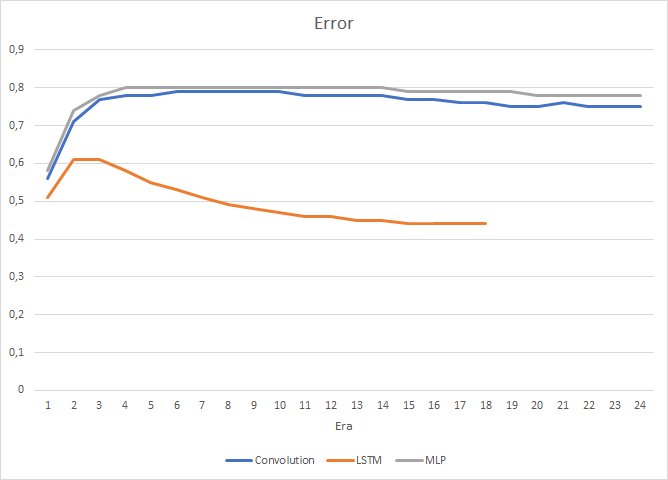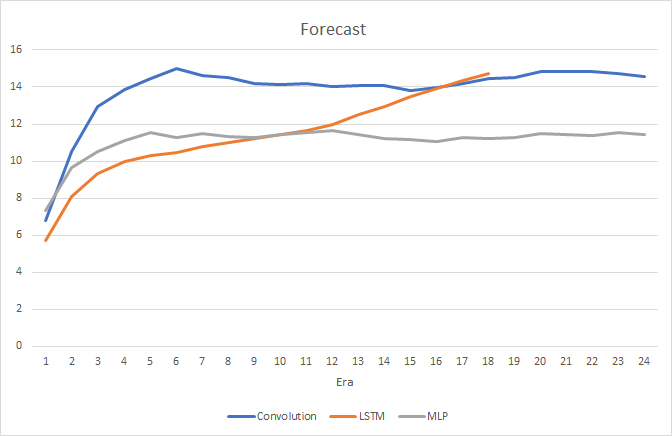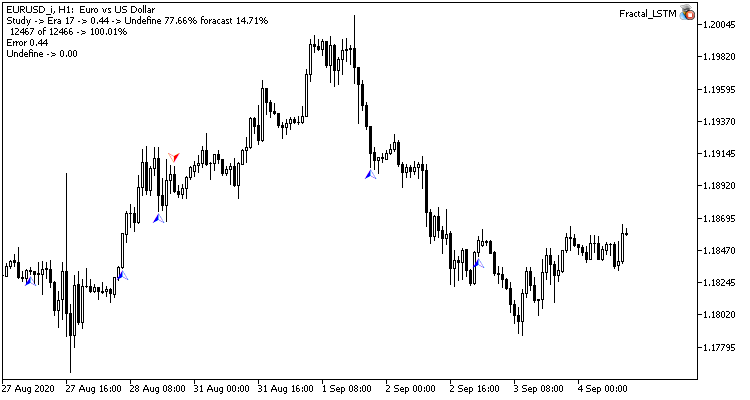### 链接

1. 神经网络变得轻松
2. 神经网络变得轻松（第二部分）：网络训练和测试
3. 神经网络变得轻松（第三部分）：卷积网络
4. 理解 LSTM 网络

### 本文中用到的程序

# 名称 类型 说明
1 Fractal.mq5   智能交易系统  一款含有回归神经网络（输出层中有 1 个神经元）的智能交易系统
2 Fractal_2.mq5  智能交易系统  一款含有分类神经网络的智能交易系统（输出层中有 3 个神经元）
3 NeuroNet.mqh  类库  创建神经网络（感知器）的类库
4 ractal_conv.mq5  智能交易系统  含有卷积神经网络（输出层中 3 个神经元）的神经智能交易系统
5 Fractal_LSTM.mq5   智能交易系统  含有循环神经网络的智能交易系统（输出层中有 3 个神经元）

## 量化课程

移动端课程